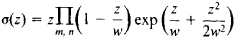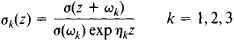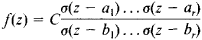Sigma Functions

The following article is from The Great Soviet Encyclopedia (1979). It might be outdated or ideologically biased.

Sigma Functions

entire transcendental functions introduced by K. Weierstrass in his theory of elliptic functions. The principal sigma function (there are four in all) iswhere w = 2mω1+ 2nω21 and ω2 are two numbers such that ω12 is not real) and m and n independently run through all positive and negative integers, other than m = n = 0. The function σ(z) has simple zeros when z = w— that is, at the vertices of the parallelograms forming a regular lattice in the z-plane. These parallelograms are obtained from the parallelogram with vertices at the points 0, 2ω1, 2ω2, and 2(ω1 + ω2) by means of translations along its sides.

The functionσ(z) can be used to determine the Weierstrass zeta function ζ(z) and the Weierstrass elliptic function ℘(z):Let ω3 = – ω1 – ω2 and ζ(ωk) = ηK, where k = 1, 2, 3. The formulas

σ(z + 2wk) = – σ(z) exp [2ηk(z + wk)] k = 1, 2, 3

express the property of quasi periodicity of the function σ(z). The equationsdefine the three remaining sigma functions. We have σ(0) = 0 and σk(0) = 1, k = 1, 2, 3. The function σ(z) is odd, and the other sigma functions are even.

Any elliptic function f(z) with periods 2ω1, and 2ω2 can be rationally expressed in terms of sigma functions by means of the formulawhere C is a constant and a1 …, a, and b1, …, br are, respectively, complete systems of zeros and poles of f(z) satisfying the condition a1 + … + ar = b1 + … + br. Sigma functions are closely related to theta functions.

REFERENCES

Smirnov, V. I. Kurs vysshei matematiki, 8th ed., vol. 3, part 2. Moscow, 1969.
Hurwitz, A., and R. Courant. Teoriia funktsii. Moscow, 1968. (Translated from German.)
Whittaker, E. T., and G. N. Watson. Kurs sovremennogo analiza, 2nd ed., part 2. Moscow, 1963. (Translated from English.)